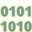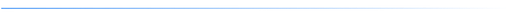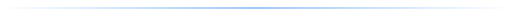2021.07.27

### A super easy way to access bit fields in JavaA bit field is a series of bits in a primitive value like an int or a long that, taken together, can hold a value. For example, display colors are represented with bit fields for red, green, blue and alpha:

``````AAAAAAAA RRRRRRRR GGGGGGGG BBBBBBBB
``````

The code required to get and set fields of a primitive involve shifting and masking operations which can be error prone and sometimes hard to follow.#### Bit diagrams

KivaKit provides the BitDiagram class to make this task easy. Taking our example of ARGB colors, we can create a BitDiagram for colors:

``````private static BitDiagram COLOR = new BitDiagram
("AAAAAAAA RRRRRRRR GGGGGGGG BBBBBBBB");
``````

In this diagram, the ‘A’ characters represent alpha bits, the ‘R’ characters represent red bits, etc. (spaces in the diagram are ignored). Given the bit diagram COLOR, we can retrieve a BitField for each field in the bit diagram by calling field(char):

``````private static BitField ALPHA = COLOR.field('A');
private static BitField RED   = COLOR.field('R');
private static BitField GREEN = COLOR.field('G');
private static BitField BLUE  = COLOR.field('B');
``````

These BitField objects contain the shift and mask values, determined from the diagram, needed to retrieve the field values from a primitive.#### Example

In this code:

``````var rgb   = 0xff8040;

var red   = RED.getInt(rgb);
var green = GREEN.getInt(rgb);
var blue  = BLUE.getInt(rgb);
``````

the BitFields RED, GREEN and BLUE are used to extract the *red, green and blue values from the rgb integer variable. After executing this code, the variable red will be 0xff, green will be 0x80 and blue will be 0x40.

We can use the same BitField objects again to set the bit fields of a primitive value:

``````rgb = RED.set(rgb, 0x12);
rgb = GREEN.set(rgb, 0x34);
rgb = BLUE.set(rgb, 0x56);
``````

After executing this code, our rgb variable will be 0x123456.#### Code

The handy BitDiagram class is in the kivakit-kernel module in KivaKit:

``````<dependency>
<groupId>com.telenav.kivakit</groupId>
<artifactId>kivakit-kernel</artifactId>
<version>\${kivakit.version}</version>
</dependency>
``````Questions? Comments? Tweet yours to @OpenKivaKit or post here: